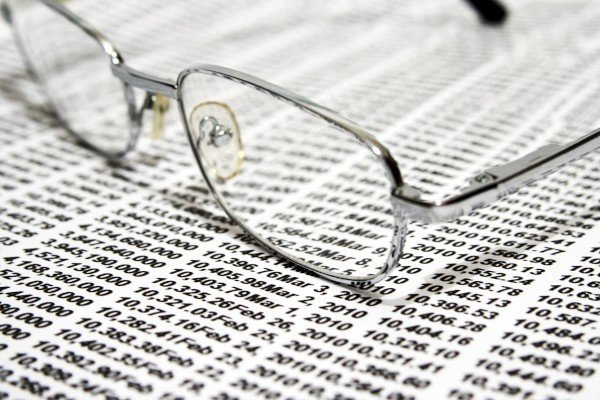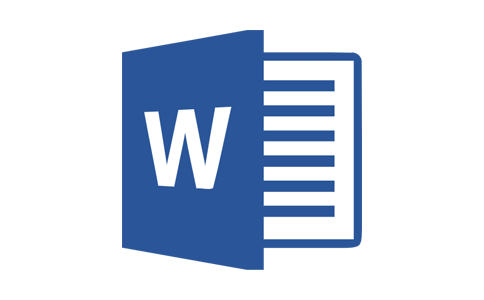# 初一年级下二元一次方程练习题苏教版1．下面为二元一次方程的是 （ ）
A．x+3y B．x+y2=0 C．x+y=2x D．x+x2=6
2．下面说法正确的是 （ ）
A．二元一次方程的解是的 ．
B．二元一次方程有无数个解．
C．二元一次方程中有一个未知数．
D．二元一次方程中的二元是指未知数的项的次数为二次．
3．下列哪组是二元一次方程2a+3b=8的一个解 ( )
A．a=1,b=2 B．a=1,b=1 C．a=2 ,b=1 D．a=2,b=2
4． 小红用20元买了3只铅笔和1和文具盒，求铅笔和文具盒的单价.设铅笔的单价为x元，文具盒的单价为y元，则可列出什么方程 （ ）
A． y-3x=20 B.3x+y=20 C.3y+x=20 D.3x-y=20

5．已知二元一次方程3x+y=0，当x=1时，y=___．
6．已知对于x、y的二元一次方程mx+nyn +（m-1）z=0，则m=，n=．
7．写出二元一次方程2a+3b=6的一个解： a=，b=．(只需填写一组你认为合适的数字即可)．

8. 根据题意列出方程：
（1）买5㎏苹果和3㎏香蕉共需30元，分别求出苹果和香蕉的单价．

（2）七年级二班男生人数的2倍比女生人数的3倍少10人，求男、女生的人数．

9. 已知二元一次方程3a+6b=12．
（1）用 含有a的式子表示b；
（2）计算当a=0,2,4时对应的b值.

10. 已知二元一次方程6x+6=3y.
（1）根据给出的x值，求出对应的y值，填入表内：
x -2 -1 0 1 2 3
y
（2）写出6x+6=3y的6个解.

1. C
【解析】二元一次方程是指有两个未知数，并且未知数的项的次数为一次的方程，A选项没有“=”号，不是；B选项y的次数为2不是1，不是；C选项有x和y两个未知数其次数都是1，是；D选项只有一个未知数，不是.
2. B
【解析】对于二元一次方程，当有一个未知数x值确定具有另一个未知数y的值与之对应，一个x值和一个对应的y组成二元一次方程的一个解。x可以取无数个，这样的解也就有无数个，所以正确答案为B.
3. A
【解析】当a=1时，带入方程得到2+3b=8，解得b=2，A答案正确，B答案错误；当a=2时，带入方程得到2×2+3b=8，解得b=，C、D答案错误.
4. C
【解析】根据20=铅笔的总价+文具盒的总价，可以得到20=3×铅笔单价+文具盒单价，根据所设的未知数可以得到20=3x+y；因此正确答案为C.

5、-3
【解析】当x=1时，方程变化为3×1+b=0，解得b=-3.
6、1；1
【解析】
∵mx+nyn +（m-1）z=0为二元一次方程
∴n=1且m-1=0
∴n=1，m=1
7、3；0（答案不）
【解析】

8、 （1）解：根据题意可列方程：
5x+3y=30
（2） 解：根据题意可列方程：
2x=3y-10
9、 （1）3a+6b=12

∴b=2- a
（2）当a=0时，带入方程得到0×3+6b=12，解得b=2；

10、解：（1）当x=-2时，带入方程得到(-2)×6+6=3y，解得y=2；

x -2 -1 0 1 2 3
y 2 0 2 4 6 8
（2）根据（1）可得，方程的解可以为：
①x=-2；y=2；
②x=-1；y=0；
③x=0；y=2；
④x=1；y=4；
⑤x=2；y=6.（答案不，只要这组x和y值使等式成立即可）Top